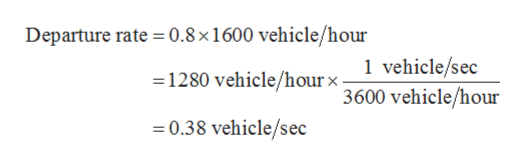# TRANSPORTATION ENGINEERING-TRAFFIC SIGNALS"Recent analysis at an approach to pre-time signalized intersection indicate that the volume-to-capacity ratio (v/c) is 0.8, htat saturation flow rate (s) is 1600 vehicles/hour, and the effective green time is 50 seconds. If the average delay, assuming D/D/1 is 11.25 seconds per vehicle, determine the arrival flow rate (vehicles/hour) and the cycle length.

Question
18 views

TRANSPORTATION ENGINEERING-TRAFFIC SIGNALS"

Recent analysis at an approach to pre-time signalized intersection indicate that the volume-to-capacity ratio (v/c) is 0.8, htat saturation flow rate (s) is 1600 vehicles/hour, and the effective green time is 50 seconds. If the average delay, assuming D/D/1 is 11.25 seconds per vehicle, determine the arrival flow rate (vehicles/hour) and the cycle length.

check_circle

Step 1

Given data:

The volume to capacity ratio (v/c) is 0.8.

The saturate flow rate (s) is 1600 vehicles/hour.

The effective green time (gi) is 50 seconds.

The average delay is 11.25 seconds per vehicle.

Step 2

The departure rate is given by,

Step 3

Plugging val...help_outlineImage TranscriptioncloseDeparture rate 0.8 x 1 600 vehicle/hour 1 vehicle/sec 3600 vehicle/hour 1280 vehicle/hourx- 0.38 vehicle/sec fullscreen

### Want to see the full answer?

See Solution

#### Want to see this answer and more?

Solutions are written by subject experts who are available 24/7. Questions are typically answered within 1 hour.*

See Solution
*Response times may vary by subject and question.
Tagged in

### Transportation Engineering